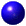LEGACY CONTENT. If you are looking for Voteview.com, PLEASE CLICK HERE

This site is an archived version of Voteview.com archived from University of Georgia on May 23, 2017. This point-in-time capture includes all files publicly linked on Voteview.com at that time. We provide access to this content as a service to ensure that past users of Voteview.com have access to historical files. This content will remain online until at least January 1st, 2018. UCLA provides no warranty or guarantee of access to these files.

45-734 Probability and Statistics II (4th Mini AY 1997-98 Flex-Mode and Flex-Time)

Assignment #2: Due 31 March 1998

1. To assist you in becoming familiar with simple linear regression, it will be necessary for you to perform the calculations in this problem by hand. Suppose you are given the following data:
```
Xi     Yi
----------
0     -2
1     -1
2      1
3      1
4      1
```
Note: In doing these calculations be careful how much rounding you do! This will become important later. (Keep, say, three significant digits.)

1. Calculate the ki values for each observation, that is (as in the Epple Notes),
```                       _               _
ki = (Xi - Xn )/[åi=1,n(Xi - Xn )2]
```
and demonstrate that:
```
åi=1,nki = 0, åi=1,nkixi = 1, and
_
åi=1,nki2 = 1/[åi=1,n(xi - Xn)2]
```
2. ```                                   ^      ^
Calculate the estimated parameters b0 and b1 for the linear
model. Plot the data by hand as well as your estimated
regression equation.```
3. For each observation xi, obtain point estimates of yi using your estimated regression equation. Demonstrate that
```                                    ^
åi=1,n yi  = åi=1,n yi
```
4. For each observation, calculate the estimated error ei. Show that åi=1,nei = 0 and
åi=1,neixi = 0. Calculate the deviation of the observations from their mean:
```                         _
(yi  - y)
```
for each yi.

5. Using the values from (d), Calculate the sum of the squared error (SSE), the total sum of squares (TSS), and the R2 of the regression.

6. ```                   ^            ^
Calculate s2, VAR(b0), and VAR(b1) for this example.
```
7. Perform the hypothesis test:

Ho: b0 = 0
H1: b0 ¹ 0.

Do the same test for b1. Find the 2-tailed P-values for both of these tests. (You can use EVIEWS to do this with the @TDIST(,) command. (See the "Using EVIEWS" handout for an example.)

2. Suppose we are in charge of a large manufacturing facility which is heated by burning a mixture of fuel oil and coal. We have records relating average hourly temperature and the tonnage of fuel consumed by our heating plant for 8 randomly chosen weeks last year. The data are
```
Average Hourly Temperature      Tons of Fuel Consumed
xi                           yi
----                         ----
28.0                         12.4
28.0                         11.7
32.5                         12.4
39.0                         10.8
45.9                          9.4
57.8                          9.5
58.1                          8.0
62.5                          7.5
```
These data are in the workfileFuel.wf1.

1. Use EVIEWS to estimate the simple linear regression of tons of fuel consumed as a function of the average hourly temperature.

2. Plot the data and the function. How well do the data fit the equation? Interpret your regression coefficients and hand in the plots.

3. Obtain a point estimate of tons of fuel consumed (i.e., the expected value) for an average hourly temperature of 41 degrees.

4. Obtain the 95 percent confidence interval for the point estimate in (c) and compute the confidence limits.

3. Suppose we are in charge of a windmill farm for an electric utility. We have records relating Wind Velocity (measured in miles per hour) against direct current output of the windmill. The data are
```
Wind Velocity                 DC Output
xi                            yi
----                         -----
2.45                         0.123
2.70                         0.500
2.90                         0.653
3.05                         0.558
3.40                         1.057
3.60                         1.137
3.95                         1.144
4.10                         1.194
4.60                         1.562
5.00                         1.582
5.45                         1.501
5.80                         1.737
6.00                         1.822
6.20                         1.866
6.35                         1.930
7.00                         1.800
7.40                         2.088
7.85                         2.179
8.15                         2.166
8.80                         2.112
9.10                         2.303
9.55                         2.294
9.70                         2.386
10.00                         2.236
10.20                         2.310
```
These data are in the workfilewindmill.wf1.

1. Use EVIEWS to estimate the simple linear regression of DC Output as function of the Wind Velocity.

2. Interpret the graph of the residuals. Do the residuals look random to you?

4. Suppose we are in charge of a papermill for a large paper company. We have records relating of the concentration of hardwoods in batches of pulp against the the tensile strength (in psi) of the paper that was manufactured from the pulp. The data are
```
Hardwood Concentration           Tensile Strength
xi                            yi
----                         -----
1.0                           6.3
1.5                          11.1
2.0                          20.0
3.0                          24.0
4.0                          26.1
4.5                          30.0
5.0                          33.8
5.5                          34.0
6.0                          38.1
6.5                          39.9
7.0                          42.0
8.0                          46.1
9.0                          53.1
10.0                          52.0
11.0                          52.5
12.0                          48.0
13.0                          42.8
14.0                          27.8
15.0                          21.9
```
These data are in the workfilehardwood.wf1.

1. Use EVIEWS to estimate the simple linear regression of Tensile Strength as function of the amount of Hardwood in the pulp.

2. Interpret the graph of the residuals. Do the residuals look random to you?